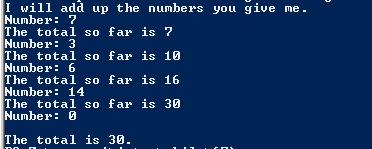# Assignment #67 and Adding Values in a loop

## Code

```    ///Name: Tim Gibson
///Period: 6
///Project Name: Adding Values in a loop
///Date Finished: 10/21/15

import java.util.Scanner;

{
public static void main ( String[] args )
{
Scanner keyboard = new Scanner(System.in);

int num, total;

total = 0;

System.out.println( "I will add up the numbers you give me." );
System.out.print( "Number: " );
num = keyboard.nextInt();
total = total + num;

while ( num != 0 )
{
System.out.println( "The total so far is " + total );
System.out.print( "Number: " );
num = keyboard.nextInt();
total = total + num;
}

System.out.println( "\nThe total is " + total + "." );
}
}
```

### Picture of the output